Function Repository Resource:

# ToTensor

Convert an expression involving Dot, Tr and MatrixPower into an equivalent expression using TensorContract

Contributed by: Carl Woll
 ResourceFunction["ToTensor"][expr] converts Dot,Tr and MatrixPower subexpressions into equivalent forms using TensorContract.

## Details and Options

ResourceFunction["ToTensor"] makes no assumptions about the rank of the symbolic tensors involved. Use the Assumptions option or \$Assumptions to define rank information for the involved tensors.
The input and output of ResourceFunction["ToTensor"] will evaluate to the same array when given explicit arrays as input.
Only Tr expressions representing traces of the first two levels of an array have equivalent TensorContract representations.

## Examples

### Basic Examples (4)

Specify the properties of symbolic arrays:

 In:=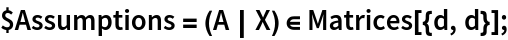A tensor built using Tr and Dot:

 In:=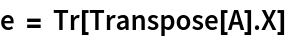Out=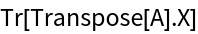Convert to an equivalent form using TensorContract:

 In:=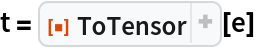Out=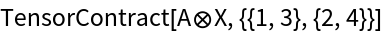Check:

 In:=Out=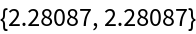### Scope (2)

Check equivalence of forms, assuming the two matrices A and B are square:

 In:=Out=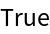A tensor consisting of Dot, Tr and MatrixPower:

 In:=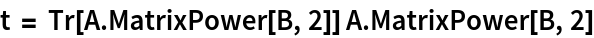Out=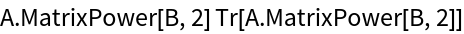Evaluate, assuming the two matrices are square:

 In:=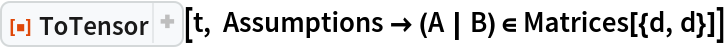Out=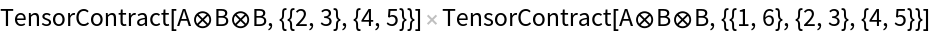### Applications (2)

TensorReduce is unable to reduce some mixed expressions consisting of both TensorContract and Dot:

 In:=Out=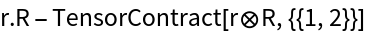Using ToTensor trivially simplifies the expression:

 In:=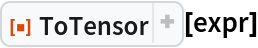Out=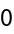### Possible Issues (2)

Rank information for symbolic tensors must be specified using the Assumptions option or using \$Assumptions:

 In:=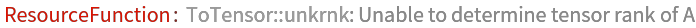Out=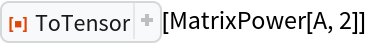Here the assumptions are added correctly:

 In:=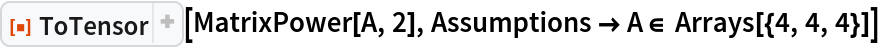Out=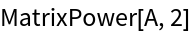Only Tr expression representing traces of the first two levels of an array have equivalent TensorContract representations:

 In:=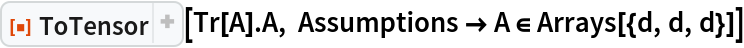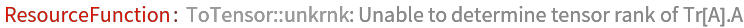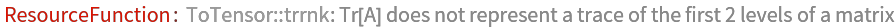Out=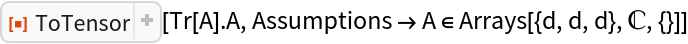Carl Woll

## Version History

• 1.1.0 – 14 December 2021
• 1.0.0 – 16 October 2019